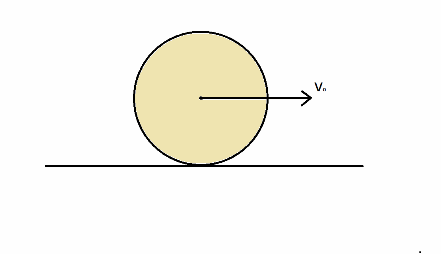# The story of a mercilessly thrown sphere...A spherical ball of mass M and radius R is thrown along a rough horizontal surface so that initially, it slides with a linear speed $\displaystyle{\upsilon_0}$,but does not rotate. As it slides, it begins to spin and eventually rolls without slipping. The time taken to start rolling can be expressed as $\dfrac{a}{b} \times \dfrac{\upsilon_0}{\mu_k g}$ Where $\displaystyle{\mu_k}$ is the coefficient of kinetic friction between the surface and the ball .What is $\displaystyle{a+b}$?

Liked it? try some more

×

Problem Loading...

Note Loading...

Set Loading...